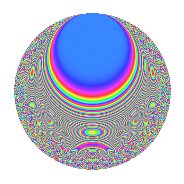Properties

 Label 4031.2.a.dLevel 4031 Weight 2 Character orbit 4031.a Self dual Yes Analytic conductor 32.188 Analytic rank 0 Dimension 98 CM No

Related objects

Newspace parameters

 Level: $$N$$ = $$4031 = 29 \cdot 139$$ Weight: $$k$$ = $$2$$ Character orbit: $$[\chi]$$ = 4031.a (trivial)

Newform invariants

 Self dual: Yes Analytic conductor: $$32.1876970548$$ Analytic rank: $$0$$ Dimension: $$98$$ Fricke sign: $$-1$$ Sato-Tate group: $\mathrm{SU}(2)$

$q$-expansion

The dimension is sufficiently large that we do not compute an algebraic $$q$$-expansion, but we have computed the trace expansion.

 $$\operatorname{Tr}(f)(q) =$$ $$98q$$ $$\mathstrut +\mathstrut 6q^{2}$$ $$\mathstrut +\mathstrut 6q^{3}$$ $$\mathstrut +\mathstrut 116q^{4}$$ $$\mathstrut +\mathstrut q^{5}$$ $$\mathstrut +\mathstrut 7q^{6}$$ $$\mathstrut +\mathstrut 12q^{7}$$ $$\mathstrut +\mathstrut 15q^{8}$$ $$\mathstrut +\mathstrut 136q^{9}$$ $$\mathstrut +\mathstrut O(q^{10})$$ $$\operatorname{Tr}(f)(q) =$$ $$98q$$ $$\mathstrut +\mathstrut 6q^{2}$$ $$\mathstrut +\mathstrut 6q^{3}$$ $$\mathstrut +\mathstrut 116q^{4}$$ $$\mathstrut +\mathstrut q^{5}$$ $$\mathstrut +\mathstrut 7q^{6}$$ $$\mathstrut +\mathstrut 12q^{7}$$ $$\mathstrut +\mathstrut 15q^{8}$$ $$\mathstrut +\mathstrut 136q^{9}$$ $$\mathstrut +\mathstrut 16q^{10}$$ $$\mathstrut +\mathstrut 25q^{11}$$ $$\mathstrut +\mathstrut 8q^{12}$$ $$\mathstrut +\mathstrut 18q^{13}$$ $$\mathstrut +\mathstrut 34q^{14}$$ $$\mathstrut +\mathstrut 14q^{15}$$ $$\mathstrut +\mathstrut 136q^{16}$$ $$\mathstrut +\mathstrut 35q^{17}$$ $$\mathstrut +\mathstrut 20q^{18}$$ $$\mathstrut +\mathstrut 48q^{19}$$ $$\mathstrut -\mathstrut 20q^{20}$$ $$\mathstrut +\mathstrut 62q^{21}$$ $$\mathstrut +\mathstrut 32q^{22}$$ $$\mathstrut +\mathstrut q^{23}$$ $$\mathstrut +\mathstrut 8q^{24}$$ $$\mathstrut +\mathstrut 173q^{25}$$ $$\mathstrut +\mathstrut 15q^{26}$$ $$\mathstrut +\mathstrut 18q^{27}$$ $$\mathstrut +\mathstrut 37q^{28}$$ $$\mathstrut +\mathstrut 98q^{29}$$ $$\mathstrut -\mathstrut 6q^{30}$$ $$\mathstrut +\mathstrut 20q^{31}$$ $$\mathstrut +\mathstrut 16q^{32}$$ $$\mathstrut +\mathstrut 24q^{33}$$ $$\mathstrut -\mathstrut 6q^{34}$$ $$\mathstrut +\mathstrut 11q^{35}$$ $$\mathstrut +\mathstrut 155q^{36}$$ $$\mathstrut +\mathstrut 62q^{37}$$ $$\mathstrut -\mathstrut 5q^{38}$$ $$\mathstrut +\mathstrut 45q^{39}$$ $$\mathstrut +\mathstrut 38q^{40}$$ $$\mathstrut +\mathstrut 50q^{41}$$ $$\mathstrut +\mathstrut 7q^{42}$$ $$\mathstrut +\mathstrut 72q^{43}$$ $$\mathstrut +\mathstrut 92q^{44}$$ $$\mathstrut -\mathstrut 13q^{45}$$ $$\mathstrut +\mathstrut 32q^{46}$$ $$\mathstrut -\mathstrut 16q^{47}$$ $$\mathstrut -\mathstrut 13q^{48}$$ $$\mathstrut +\mathstrut 194q^{49}$$ $$\mathstrut +\mathstrut 50q^{50}$$ $$\mathstrut +\mathstrut 2q^{51}$$ $$\mathstrut +\mathstrut 83q^{52}$$ $$\mathstrut -\mathstrut 11q^{53}$$ $$\mathstrut +\mathstrut 58q^{54}$$ $$\mathstrut +\mathstrut 32q^{55}$$ $$\mathstrut +\mathstrut 106q^{56}$$ $$\mathstrut +\mathstrut 84q^{57}$$ $$\mathstrut +\mathstrut 6q^{58}$$ $$\mathstrut +\mathstrut 20q^{59}$$ $$\mathstrut +\mathstrut 62q^{60}$$ $$\mathstrut +\mathstrut 142q^{61}$$ $$\mathstrut -\mathstrut 27q^{62}$$ $$\mathstrut +\mathstrut 26q^{63}$$ $$\mathstrut +\mathstrut 213q^{64}$$ $$\mathstrut +\mathstrut 13q^{65}$$ $$\mathstrut -\mathstrut 35q^{66}$$ $$\mathstrut +\mathstrut 5q^{67}$$ $$\mathstrut +\mathstrut 69q^{68}$$ $$\mathstrut +\mathstrut 68q^{69}$$ $$\mathstrut -\mathstrut 2q^{70}$$ $$\mathstrut -\mathstrut 10q^{71}$$ $$\mathstrut -\mathstrut 9q^{72}$$ $$\mathstrut +\mathstrut 74q^{73}$$ $$\mathstrut +\mathstrut 21q^{74}$$ $$\mathstrut +\mathstrut 19q^{75}$$ $$\mathstrut +\mathstrut 116q^{76}$$ $$\mathstrut +\mathstrut 41q^{77}$$ $$\mathstrut -\mathstrut 162q^{78}$$ $$\mathstrut +\mathstrut 104q^{79}$$ $$\mathstrut -\mathstrut 86q^{80}$$ $$\mathstrut +\mathstrut 230q^{81}$$ $$\mathstrut +\mathstrut 53q^{82}$$ $$\mathstrut -\mathstrut 19q^{83}$$ $$\mathstrut +\mathstrut 76q^{84}$$ $$\mathstrut +\mathstrut 125q^{85}$$ $$\mathstrut +\mathstrut 9q^{86}$$ $$\mathstrut +\mathstrut 6q^{87}$$ $$\mathstrut +\mathstrut 68q^{88}$$ $$\mathstrut +\mathstrut 120q^{89}$$ $$\mathstrut +\mathstrut 122q^{90}$$ $$\mathstrut +\mathstrut 30q^{91}$$ $$\mathstrut +\mathstrut 3q^{92}$$ $$\mathstrut -\mathstrut 56q^{93}$$ $$\mathstrut +\mathstrut 22q^{94}$$ $$\mathstrut +\mathstrut 32q^{95}$$ $$\mathstrut -\mathstrut 55q^{96}$$ $$\mathstrut +\mathstrut 98q^{97}$$ $$\mathstrut -\mathstrut 15q^{98}$$ $$\mathstrut +\mathstrut 69q^{99}$$ $$\mathstrut +\mathstrut O(q^{100})$$

Embeddings

For each embedding $$\iota_m$$ of the coefficient field, the values $$\iota_m(a_n)$$ are shown below.

For more information on an embedded modular form you can click on its label.

Label $$a_{2}$$ $$a_{3}$$ $$a_{4}$$ $$a_{5}$$ $$a_{6}$$ $$a_{7}$$ $$a_{8}$$ $$a_{9}$$ $$a_{10}$$
1.1 −2.78351 2.95190 5.74795 −3.19044 −8.21665 −4.40114 −10.4325 5.71371 8.88064
1.2 −2.76750 0.603367 5.65907 3.54828 −1.66982 1.63115 −10.1265 −2.63595 −9.81988
1.3 −2.75511 −2.40315 5.59062 −0.846757 6.62092 3.63959 −9.89254 2.77511 2.33291
1.4 −2.74332 −0.716173 5.52580 −3.18087 1.96469 1.27957 −9.67240 −2.48710 8.72614
1.5 −2.61634 −3.34235 4.84525 −3.10216 8.74473 −4.97241 −7.44414 8.17130 8.11632
1.6 −2.59643 0.964956 4.74146 3.18498 −2.50544 −0.304804 −7.11802 −2.06886 −8.26958
1.7 −2.58392 3.19893 4.67665 1.67511 −8.26577 1.22424 −6.91625 7.23312 −4.32836
1.8 −2.57508 1.48970 4.63103 −2.75202 −3.83609 2.46323 −6.77510 −0.780799 7.08668
1.9 −2.48847 −3.00036 4.19251 0.297405 7.46633 −2.60645 −5.45599 6.00219 −0.740084
1.10 −2.44621 0.244458 3.98396 −3.48270 −0.597997 1.44143 −4.85320 −2.94024 8.51943
1.11 −2.43943 −2.23361 3.95082 1.05551 5.44873 −1.84487 −4.75890 1.98900 −2.57484
1.12 −2.22708 1.27662 2.95990 0.197788 −2.84313 −3.89763 −2.13778 −1.37025 −0.440491
1.13 −2.11438 2.42067 2.47061 1.02780 −5.11822 0.853130 −0.995051 2.85965 −2.17316
1.14 −2.08450 0.675318 2.34516 −1.05546 −1.40770 −4.46025 −0.719483 −2.54395 2.20012
1.15 −2.03151 −1.34287 2.12703 3.37426 2.72805 0.525143 −0.258068 −1.19670 −6.85484
1.16 −1.99223 −2.07417 1.96900 0.414014 4.13224 4.11338 0.0617619 1.30220 −0.824814
1.17 −1.97068 0.422540 1.88358 2.63171 −0.832693 −1.79791 0.229417 −2.82146 −5.18627
1.18 −1.96419 −0.913998 1.85806 −4.29424 1.79527 −2.01487 0.278799 −2.16461 8.43472
1.19 −1.93664 0.437969 1.75056 3.14696 −0.848187 5.11562 0.483081 −2.80818 −6.09452
1.20 −1.86328 0.307270 1.47182 0.176749 −0.572530 −0.402020 0.984149 −2.90559 −0.329334
See all 98 embeddings
 $$n$$: e.g. 2-40 or 990-1000 Embeddings: e.g. 1-3 or 1.98 Significant digits: Format: Complex embeddings Normalized embeddings Satake parameters Satake angles

Inner twists

This newform does not have CM; other inner twists have not been computed.

Atkin-Lehner signs

$$p$$ Sign
$$29$$ $$-1$$
$$139$$ $$1$$

Hecke kernels

This newform can be constructed as the kernel of the linear operator $$T_{2}^{98} - \cdots$$ acting on $$S_{2}^{\mathrm{new}}(\Gamma_0(4031))$$.